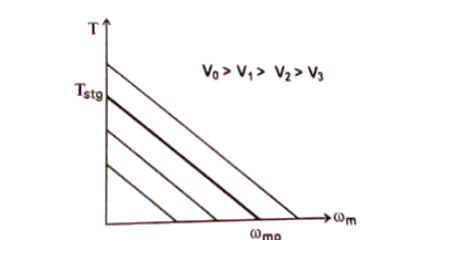Home | | Special Electrical Machines | Torque- Speed Charactersistics of BLPM SQM DC Motor

# Torque- Speed Charactersistics of BLPM SQM DC Motor

Let the supply voltage V be constant. A family of torque speed characteristics for various constant supply voltages is as shown in figure 4.20

TORQUE- SPEED CHARACTERSISTICS OF BLPM SQM DC MOTOR

Let the supply voltage V be constant. A family of torque speed characteristics for various constant supply voltages is as shown in figure 4.20Fig 4.20 T-Ď‰m curve for various supply voltages

Permissible region of operation in T-Ď‰m plane

Torque speed characteristics of BLPM square wave motor is shown in fig.4.21. The constraints are

1.     The continues current should not exceed the permissible current limit In (i.e) Torques should not exceed Kt In.

2.     The maximum permissible supply voltage = Vn.

3.     The speed should not exceed Ď‰mn.Line AB

Parallel to X-axis represents maximum permissible torque line which corresponds to maximum permissible current In.

Line FG

It represents T-Ď‰m characteristics corresponding to the maximum permissible Vn. B and C are points in Fg. B is the point of intersection between AB and FG.

Line DH

It represents constant maximum permissible speed line (i.e) Ď‰mn is constant. DH intersects FG and x axis at D.

The area OABCDO is the permissible region of operation. To obtain a particular point P corresponding to given load-torque and speed condition the only way to operate the motor at P is by suitably adjusting the supply voltage fed to the motor.v   If the phase resistance is small as it should be in an efficient design, then the characteristics to that of a shunt dc motor. The speed is essentially controlled by the voltage V and may be changed by changing the supply voltage. Then the current drawn just to drive the torque at its speed.

v   As the load torque is increased, the speed drops and the drop is directly proportional to the phase resistance and the torque.

v   The voltage is usually controlled by chopping or PWM. This gives rise to a family of torque speed characteristics as shown in fig. 4.22. The boundaries of continuous and intermittent limits are shown.

Continuous limit - determined by the heat transfer and temperature rise.

Intermittent limit â€“ determined by the maximum ratings of semiconductor devices in circuit.

In practice the torque speed characteristics deviates from the ideal form because of the effects of inductance and other parasitic influences.

Also the speed range can be extended by increasing the dwell of conduction period relative to the rotor position.

Study Material, Lecturing Notes, Assignment, Reference, Wiki description explanation, brief detail
Special Electrical Machines : Permanent Magnet Brushless D.C. Motors : Torque- Speed Charactersistics of BLPM SQM DC Motor |